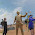VHDL coding tips and tricks: Synchronous Vs Asynchronous resets in VHDL

## Tuesday, April 13, 2010

### Synchronous Vs Asynchronous resets in VHDL

In your design you may need to initialize all your signals to a predetermined state.This is done by applying a reset signal.A reset signal can change the system in two ways: Synchronous and asynchronous.In this article I have tried to explain the advantages and disadvantages of both the methods and how exactly it is implemented in hardware.

Synchronous Reset :

A synchronous reset signal can be applied as shown below :

process(clk)
begin
if(rising_edge(clk)) then
if(reset = '0') then  --reset is checked only at the rising edge of clock.
o <= i;
else
o <= '0';
end if;
end if;
end process;

The code is synthesised to the following block in FPGA: (truth table of FDR flip flop is also given)
In the schematic diagram FDR is a single D-type flip-flop with data (D) and synchronous reset (R) inputs and data output (Q). The synchronous reset (R) input, when High, overrides all other inputs and resets the Q output Low on the 0 to 1 clock (C) transition. The data on the D input is loaded into the flip-flop when R is Low during the 0 to1 clock transition.If you analyze the above code you can see that the value of reset changes the signal 'o' only at the rising edge of the clock.This method has the following advantages:
1)The reset applied to all the flip-flops are fully synchronized with clock and always meet the reset recovery time.
2)In some cases, synchronous reset will synthesis to smaller flip-flops.
Synchronous resets have some disadvantages also:
1)If the reset applied is for a small duration then the clock edge may not be able to capture the reset signal.Thus if you are synchronous resets make sure that your reset signal stays active for enough time so that it get captured by the clock.
2)Also the change in reset doesn't immediately reflect in the associated signals.

Asynchronous reset :

Now let us have a look at the asynchronous reset :

process(clk,reset)
begin
if(reset = '0') then  --change in reset get immediately reflected on signal 'o'.
if(rising_edge(clk)) then
o <= i;
end if;
else
o <= '0';
end if;
end process;

The code is synthesised to the following in FPGA. (the truth table of the particular flip-flop is also given)

In the schematic FDC is a single D-type flip-flop with data (D) and asynchronous clear (CLR) inputs and data output (Q). The asynchronous CLR, when High, overrides all other inputs and sets the Q output Low. The data on the D input is loaded into the flip-flop when CLR is Low on the 0 to 1 clock transition.If you analyse the code you can see that when the reset goes high , immediately signal 'o' will become '0'.It doesn't wait for clock change.Now let us look at the advantages of this method:
1)High speed can be achieved.
2)Data can be reset without waiting for the clock edge.
The disadvantages are:
1) Asynchronous resets have metastability problems. By metastability what I mean is that,the clock and reset have no relationship.So if the reset  changes from 1 to 0 at the rising edge of the clock, the output is not determinate. The reset input has to follow the reset recovery time rule.This time is a kind of setup time condition on a flip-flop that defines the minimum amount of time between the change in reset signal and the next rising clock edge.If the signal doesn't follow this set up time then it may create metastability problems.

Note :- As you can see both the methods have their own advantages and disadvantages.And selecting one of the method depends upon your design requirement.There is another method of resetting the signals known as "Asynchronous Assertion, Synchronous De-assertion" which is the best method of resetting signals.This will be discussed in the next article.

#### 3 comments:

1.the other advantage to synchronous resets is that the synchronous set (for FPGAs) can now be used by logic. Xilinx has a whitepaper on this.

a large disadvantage is that it is a large net, and can limit clock rate unless pipelined. This can be an issue for coregen/other IP.

some elements, like DSP48's, only have sync resets.

2.Does someone know about the difference in term of ressouces used for the synchronous et asynchrounous process?

3.can you interface adc804 and papilio one and then give output to a 16*2 lcd